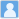• ### Majorana Neutrino Masses from Neutrinoless Double-Beta Decays and Lepton-Number-Violating Meson Decays

分类： 物理学 >> 核物理学 提交时间： 2016-09-06Jun-Hao Liu Jue Zhang Shun Zhou

摘要：The Schechter-Valle theorem states that a positive observation of neutrinoless double-beta (0νββ) decays implies a finite Majorana mass term for neutrinos when any unlikely fine-tuning or cancellation is absent. In this note, we reexamine the quantitative impact of the Schechter-Valle theorem, and find that current experimental lower limits on the half-lives of 0νββ-decaying nuclei have placed a restrictive upper bound on the Majorana neutrino mass |δmeeν|<7.43×10−29 eV radiatively generated at the four-loop level. Furthermore, we generalize this quantitative analysis of 0νββ decays to that of the lepton-number-violating (LNV) meson decays M−→M′++ℓ−α+ℓ−β (for α, β = e or μ). Given the present upper limits on these rare LNV decays, we have derived the loop-induced Majorana neutrino masses |δmeeν|<9.7×10−18 eV, |δmeμν|<1.6×10−15 eV and |δmμμν|<1.0×10−12 eV from K−→π++e−+e−, K−→π++e−+μ− and K−→π++μ−+μ−, respectively. A partial list of radiative neutrino masses from the LNV decays of D, Ds and B mesons is also given.

同行评议状态:待评议